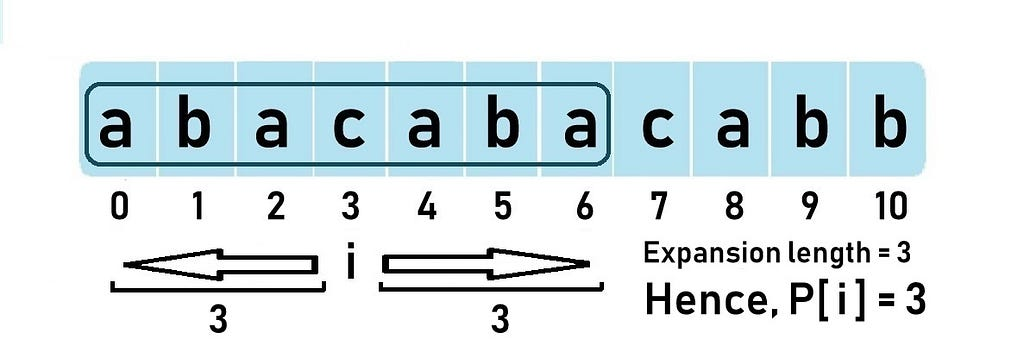# Manacher’s Algorithm Explained— Longest Palindromic Substring

Manacher’s Algorithm helps us find the longest palindromic substring in the given string. It optimizes over the brute force solution by using some insights into how palindromes work. How? Let’s see!

Let’s focus on odd length palindromes for simplicity. We go about the string from left to right.

Notations:

Let c be the center of the longest length palindrome we have encountered till now. And let l and r be the left and right boundaries of this palindrome, i.e., the left-most character index and the right-most character index respectively. Now, let’s take an example to understand c, l, and r.

Eg: “abacabacabb”

When going from left to right, when i is at index 1, the longest palindromic substring is “aba” (length = 3).

The answer for the given string is 9 when the palindrome is centered at index 5; c, l, and r are as follows:

Now that we know what c, l, and r denote, let’s take a small break from the algorithm and understand a few interesting facts about palindromes.

Mirror index: For any palindrome centered at a center c, the mirror of index j is j’ such that

For palindrome “abacaba”, the mirror of j is j’ and the mirror of j’ is j.

Now, come back to the algorithm. When we move i from left to right, we try to “expand” the palindrome at each i. When I say the word expand, it means that I’ll check whether there exists a palindrome centered at i and if there exists one, I’ll store the “expansion length” to the left or to the right in a new array called P[] array or (some prefer) LPS[].

If the palindrome at i expands beyond the current right boundary r, then c is updated to i and new l, r are found and updated.

Example time. Let’s take the previously discussed palindrome “abacaba” which is centered at i = 3.P[i] = 3 as expansion length for palindrome centered at i is 3

Therefore, the P[] array stores the expansion length of the palindrome centered at each index. But we don’t need to manually go to each index and expand to check the expansion length every time. This is exactly where Manacher’s algorithm optimizes better than brute force, by using some insights on how palindromes work. Let’s see how the optimization is done.

Important recap: c indicates the center of the current longest odd length palindrome. And l, r denote the palindrome’s left and right boundaries.

Let’s see the P[] array for the string “abacaba”.

When i = 4, the index is inside the scope of the current longest palindrome, i.e., i < r. So, instead of naively expanding at i, we want to know the minimum expansion length that is certainly possible at i, so that we can expand on that minimum P[i] and see, instead of doing from start. So, we check for mirror i’.

As long as the palindrome at index i’ does NOT expand beyond the left boundary (l) of the current longest palindrome, we can say that the minimum certainly possible expansion length at i is P[i’].

Remember that we are only talking about the minimum possible expansion length, the actual expansion length could be more and, we’ll find that out by expanding later on. In this case, P = P = 0. We try to expand but still, P remains 0.

Now, if the palindrome at index i’ expands beyond the left boundary (l) of the current longest palindrome, we can say that the minimum certainly possible expansion length at i is r-i.

Eg: “acacacb”

So, P = 5–4 = 1

You could ask but why can’t the palindrome centered at i expand after r in this case? If it did, then it would have already been covered with the current center c only. But, it didn’t. So, P[i] = r-i.

(That is, if palindrome at i were to expand beyond r, then the character at index 6 should have been ‘a’. But if that were to happen, the current palindrome centered at c would NOT have been “cacac” but “acacaca”)

So, we can sum the two quoted points above as follows:

That’s it. Now the only thing left is to expand at i after P[i], so we check characters from index (P[i] + 1) and keep expanding at i.

If this palindrome expands beyond r, update c to i and r to (i + P[i]).

Voila! The P[] array is now filled and the maximum value in this array gives us the longest palindromic substring in the given string!

We have taken an odd length string for the above explanation. So, for this algorithm to work out, we simply append N + 1 special characters (say ‘#’) in between every two characters, just to make sure that our modified string is always of odd length.

Eg1: aba -> #a#b#a#
Eg2: abba-> #a#b#b#a#

Here’s the implementation in Java:

Time Complexity: O(N)
Space Complexity: O(N)

Feel free to voice your doubts and concerns in the comments below. Thank you for reading! 😃Manacher’s Algorithm Explained— Longest Palindromic Substring was originally published in HackerNoon.com on Medium, where people are continuing the conversation by highlighting and responding to this story.